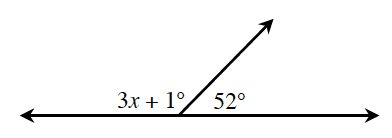### Home > CCG > Chapter 6 > Lesson 6.1.1 > Problem6-6

6-6.

Using the diagram below, write an equation and find $x$. Check your answer.What is the measure of a straight angle? How can you use that measure to find $x$?